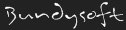L3DT users' communityLarge 3D terrain generator

## Climate: Land type score

One of the trickier subjects in L3DT

### Climate: Land type score

Hey. Great program but I'm having a little trouble working out the mathematics of land type assignments.

What is the possible numeric range of "gradient" and "curvature", etc in this calculation:

score = BaseScore + gradient × cgrad + curvature × ccurv + ... etc
[]Aba
New member

Posts: 2
Joined: Wed Apr 01, 2009 5:21 pm

Hi Aba,

The gradient can range from 0 (flat) to infinity* (vertical). For reference, 45 degrees is a gradient of 1. If you prefer to think in degrees, you can convert the gradient to a slope angle by calculating the arctangent, and vice versa by calculating the tangent.

Similarly, the curvature is the rate of change of the gradient, so it can similarly range from 0 to infinity.

Cheerio,
Aaron.

* Strictly speaking, the gradient is undefined when vertical. However, it gradient tends towards infinity as the gradient approaches vertical.Aaron

Posts: 3691
Joined: Sun Nov 20, 2005 2:41 pm
Location: Melbourne, Australia

So gradient = Horizontal component / vertical component?
[]Aba
New member

Posts: 2
Joined: Wed Apr 01, 2009 5:21 pm

Nearly, it's:

Code: Select all
`gradient = rise / run = vertical change / horizontal change`

For a nice description of gradients, please refer to the Wikipedia entry for slope. For what it's worth, gradient is the formal mathematical terms for the first spatial derivative of a scalar field (e.g. a heightfield). 'Slope' or 'grade' are the colloquial/engineering equivalents. I don't know of any colloqual/engineering equivalents for curvature (the second spatial derivative), but no matter, the formula is:

Code: Select all
`curvature = gradient change / horizontal change`

To calculate the curvature over a step, you have to calculate the gradient at both ends of your step, take the difference, then divide by the length of the horizontal step. Always takes the absolute (i.e. positive) value, since it doesn't need to care about the direction of curvature. If you're calculating the curvature at a point on the map, this means calculating the average of the X and Y curvatures, which means you have to calculate four gradients.

Note however that most land types have zero parameters for curvature. It is seldom required to achieve the desired affect.

Cheerio,
Aaron.Aaron